Electron. J. Differential Equations, Vol. 2019 (2019), No. 117, pp. 1-32.

### Initial value problems for Caputo fractional equations with singular nonlinearities Jeffrey R. L. Webb

Abstract:
We consider initial value problems for Caputo fractional equations of the form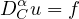where f can have a singularity. We consider all orders and prove equivalences with Volterra integral equations in classical spaces such as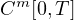. In particular for the case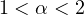we consider nonlinearities of the form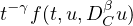where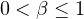and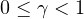with f continuous, and we prove results on existence of global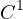solutions under linear growth assumptions on f(t,u,p) in the u,p variables. With a Lipschitz condition we prove continuous dependence on the initial data and uniqueness. One tool we use is a Gronwall inequality for weakly singular problems with double singularities. We also prove some regularity results and discuss monotonicity and concavity properties.

Submitted November 20, 2018. Published October 30, 2019.
Math Subject Classifications: 34A08, 34A12, 26A33, 26D10.
Key Words: Fractional derivatives; Volterra integral equation; weakly singular kernel; Gronwall inequality.

An addendum was posted on August 5, 2020. It provides additional information and corrects some typos. See the last page of this article.

Show me the PDF file (445 KB), TEX file for this article.Jeffrey R. L. Webb School of Mathematics and Statistics University of Glasgow Glasgow G12 8SQ, UK email: jeffrey.webb@glasgow.ac.uk